• python矩阵运算
2020-07-24 17:57:36

python矩阵运算程序

There are following matrix operations, that we can implement with the numpy matrix.

我们可以使用numpy矩阵实现以下矩阵运算

Operations that we are performing here, (Here, x and y are the matrices)...

我们在此处执行的操作(此处x和y是矩阵)...

OperationsFunctionOperator
Subtracting matrix elementssubtract(x,y)x-y
Multiplying matrix elementsmultiply(x,y)x*y
Dividing matrix elementsdivide(x,y)x/y
Product of the matricesdot(x,y)---
Square root of the matrix elementssqrt(x)---
运作方式 功能 操作员
添加矩阵元素 加(x，y) x + y
减去矩阵元素 减去(x，y) y
乘法矩阵元素 乘(x，y) x * y
划分矩阵元素 除(x，y) x / y
矩阵的乘积 点(x，y) ---
矩阵元素的平方根 sqrt(x) ---

Example:

例：

# Python program for matrix operations

# importing numpy
import numpy as np

mat1 = np.array([[10,20,30],[40,50,60],[70,80,90]])
mat2 = np.array([[1,2,3],[4,5,6],[7,8,9]])

print("mat1+mat2...")
print(mat1+mat2)
print() # prints newline

# subtracting  matrices
print("mat1-mat2...")
print(mat1-mat2)
print("np.subtract(mat1,mat2)...")
print(np.subtract(mat1,mat2))
print() # prints newline

# dividing  matrices
print("mat1/mat2...")
print(mat1/mat2)
print("np.divide(mat1,mat2)...")
print(np.divide(mat1,mat2))
print() # prints newline

# multiplying  matrices
print("mat1*mat2...")
print(mat1*mat2)
print("np.multiply(mat1,mat2)...")
print(np.multiply(mat1,mat2))
print() # prints newline

# producting matrices
print("np.dot(mat1,mat2)...")
print(np.dot(mat1,mat2))
print() # prints newline

# Square root of matrix elements
print("np.sqrt(mat1)...")
print(np.sqrt(mat1))
print() # prints newline

# Square root of matrix elements
print("np.sqrt(mat2)...")
print(np.sqrt(mat2))
print() # prints newline



Output

输出量

mat1+mat2...
[[11 22 33]
[44 55 66]
[77 88 99]]
[[11 22 33]
mat1+mat2...
[[11 22 33]
[44 55 66]
[77 88 99]]
mat1+mat2...
[[11 22 33]
[44 55 66]
[77 88 99]]
[[11 22 33]
[44 55 66]
[77 88 99]]

mat1-mat2...
[[ 9 18 27]
[36 45 54]
[63 72 81]]
np.subtract(mat1,mat2)...
[[ 9 18 27]
[36 45 54]
mat1+mat2...
[[11 22 33]
[44 55 66]
[77 88 99]]
[[11 22 33]
[44 55 66]
[77 88 99]]

mat1-mat2...
[[ 9 18 27]
[36 45 54]
[63 72 81]]
np.subtract(mat1,mat2)...
[[ 9 18 27]
[36 45 54]
[63 72 81]]

mat1/mat2...
[[10. 10. 10.]
[10. 10. 10.]
[10. 10. 10.]]
np.divide(mat1,mat2)...
[[10. 10. 10.]
[10. 10. 10.]
[10. 10. 10.]]

mat1*mat2...
[[ 10  40  90]
[160 250 360]
[490 640 810]]
np.multiply(mat1,mat2)...
[[ 10  40  90]
[160 250 360]
[490 640 810]]

np.dot(mat1,mat2)...
[[ 300  360  420]
[ 660  810  960]
[1020 1260 1500]]

np.sqrt(mat1)...
[[3.16227766 4.47213595 5.47722558]
[6.32455532 7.07106781 7.74596669]
[8.36660027 8.94427191 9.48683298]]

np.sqrt(mat2)...
[[1.         1.41421356 1.73205081]
[2.         2.23606798 2.44948974]
[2.64575131 2.82842712 3.        ]]




python矩阵运算程序python numpy tensorflow 机器学习
更多相关内容
• ## python矩阵运算

千次阅读 2022-02-01 17:23:11
矩阵m\*1可以和1\*k相乘，得到矩阵m\*k，但矩阵m\*n(n≠1)不可以和1\*k相乘(k≠n) 1.将一维行向量转化为一维列向量 注意：此处不能用a = a.T或a = np.transpose(a)来进行转置，这两种方法在a为多维矩阵时才有效 a = ...

# 1.array

## 1) 矩阵的乘法

矩阵乘法为A @ Bnp.dot(A, B)，若为对应元素相乘则用A*Bnp.multiply(A,B)

A = np.array([[1,2],[3,4]])
B = np.array([[1,2],[1,2]])
C = A @ B
D = np.dot(A, B)

输出:
C、D均为:
[[ 3  6]
[ 7 14]]


## 2) 将一维行向量转化为一维列向量

注意：python中的array若给了初始值如a = np.array([1, 2, 3])则不能用a = a.Ta = np.transpose(a)来进行转置，而用range、np.zeros()、np.ones()等函数赋予初始值可以，这两种方法在a为多维矩阵时才也有效，但matrix可以用a.T可以

a = np.array([1, 2, 3])
a = a.T

输出:[1 2 3]

a = range(4)
a = np.array([a]).T
输出:
[


]


## 3) 矩阵m*1可以和1*k相乘，得到矩阵m*k，但矩阵m*n(n≠1)不可以和1*k相乘(k≠n)

注意：此处的相乘指的是两个矩阵对应位置上的元素相乘，若想实现矩阵的相乘，可以使用np.dot()

a = np.array(range(4))
a = np.array([a]).T
b = np.array(range(3))
c = a*b

输出:
a:输出形式如上
b:[0 1 2]
c:
[[0 0 0]
[0 1 2]
[0 2 4]
[0 3 6]]


矩阵m*n(n≠1)不可以和1*k相乘(k≠n)：

a = [[1, 2],[3, 4],[5, 6]]
a = np.array(a)
b = np.array(range(3))
c = a*b		# 不能相乘

输出:
a的shape为3*2，而b的shape为(3,)，即一维数组，数组里有3个元素


矩阵m*k(n≠1)可以和1*k相乘：

a = [[1, 2],[3, 4],[5, 6]]
a = np.array(a)
b = np.array(range(2))
c = a*b		# 不能相乘

输出:
a:
[[1 2]
[3 4]
[5 6]]
b:
[0 1]
c:
[[0 2]
[0 4]
[0 6]]


# 2. matrix

## 1) 矩阵乘法

直接用A*B即可，若想实现对应元素相乘，用np.multiply(A, B)

A = np.matrix([[1,2],[1,2]])
B = np.matrix([[1,3],[2,2]])
C = A * B
D = np.multiply(A, B)

输出:
A:
[[1 2]
[1 2]]
B:
[[1 3]
[2 2]]
C:
[[5 7]
[5 7]]
D:
[[1 6]
[2 4]]


## 3) 若python中matrix为整数矩阵，对其一个元素做运算时，其运算结果会向0的方向取整

a = np.matrix([3, 2])
a[0, 0] = a[0, 0] - 0.2

输出:
a:[[2 2]]

a = np.matrix([3, 2])
a[0, 0] = a[0, 0] - 4.2

输出:
a:[[-1 2]]


由于以上特性，故若相对一个matrix的所有元素重新赋值时，可以直接用对整体赋值而不是对其内部元素一个一个地赋值，比如：
应该这样写，

theta = theta - t


而不是这样写（这样得到的结果会向零取整）:

theta[0, 0] = theta[0, 0] - t[0, 0]
theta[0, 1] = theta[0, 1] - t[0, 1]


例如：

a = np.matrix([3, 2])
c = np.matrix([0.5, 0.5])
a = a - c

输出:
a:[[2.5 1.5]]

a = np.matrix([3, 2])
c = np.matrix([0.5, 0.5])
a[0, 0] = a[0, 0] - c[0, 0]
a[0, 1] = a[0, 1] - c[0, 1]

输出:
a:[[2 1]]

展开全文python
• 主要介绍了python矩阵运算,转置,逆运算,共轭矩阵实例，具有很好的参考价值，希望对大家有所帮助。一起跟随小编过来看看吧
•Python
• Python矩阵运算 选择题 以下python代码相关知识点描述有误的一项是? import numpy as np y=np.matrix([[1,2],[3,4]]) print("y：\n",y) print("y1：\n",y.T) print("y2：\n",y.T.dot(y)) A选项：np.matrix...

【小白从小学Python、C、Java】

【Python-计算机等级考试二级】

【Python-数据分析】

Python矩阵运算

选择题

以下python代码相关知识点描述有误的一项是?

import numpy as np

y=np.matrix([[1,2],[3,4]])

print("y：\n",y)

print("y1：\n",y.T)

print("y2：\n",y.T.dot(y))

A选项：np.matrix表示生成矩阵

B选项：y.T表示y的转置矩阵

C选项：dot()函数表示矩阵的乘法

D选项：y2的结果是4行4列的矩阵

温馨期待

期待大家提出宝贵建议，互相交流，收获更大

答案

正确答案是：D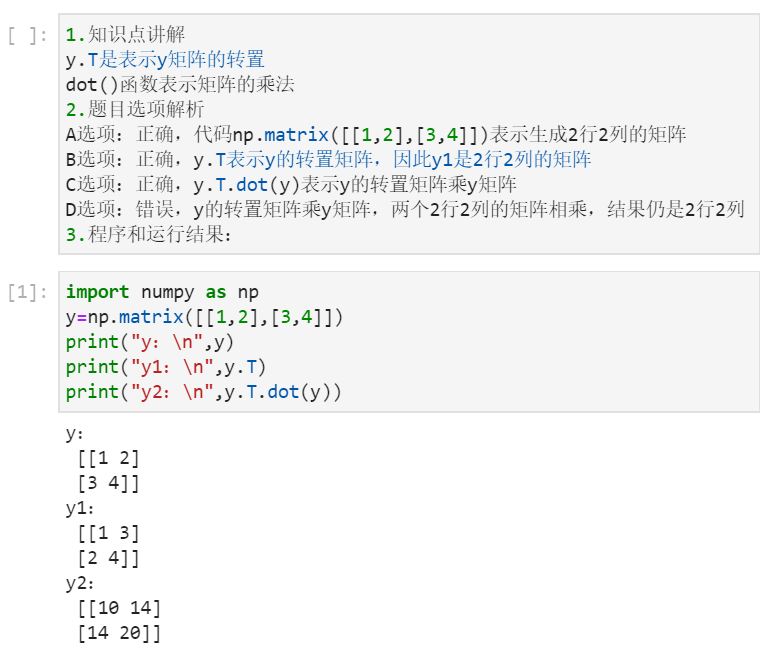展开全文python
• 机器学习数学基础之Python矩阵运算 Python矩阵基本运算 Python矩阵操作 创建矩阵与行列转换的功能函数，而在Python中也较多使用二维数组替代矩阵来进行运算 Python矩阵乘法 矩阵乘法的定律检验，要区分数乘与矩阵...

## Python矩阵基本运算

Python矩阵操作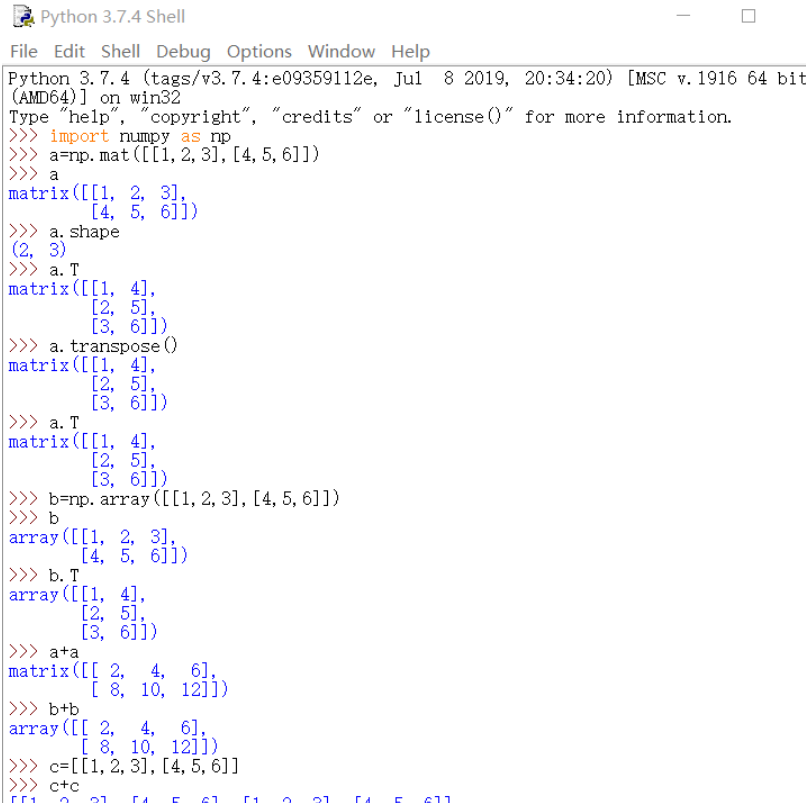创建矩阵与行列转换的功能函数，而在Python中也较多使用二维数组替代矩阵来进行运算

Python矩阵乘法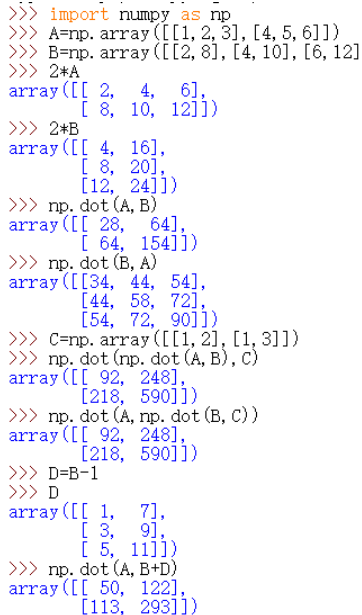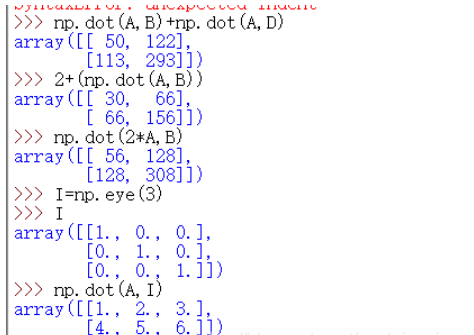矩阵乘法的定律检验，要区分数乘与矩阵相乘的情况，后者将前后次序调转也会影响结果

Python矩阵转置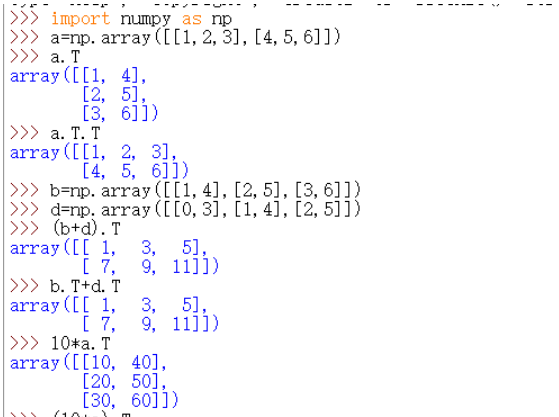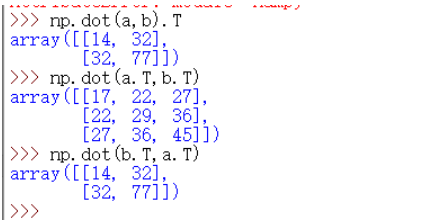矩阵转置即为行变为列、列变为行，对于转置还有一些叠加定律值得注意

Python求方阵的迹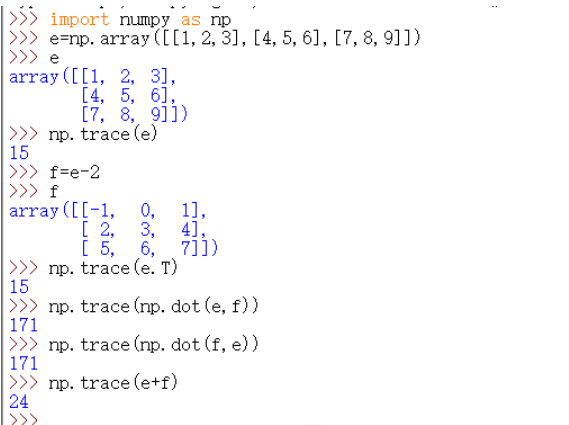方阵的迹就是主对角元素之和，也只有方阵才有迹的概念，方阵之和的迹等于方阵之迹的和

Python方针的行列式计算方法

首先引入行列式的计算概念,手工计算比较困难，但在Python中只需要运用linalg.det()函数便可简便运算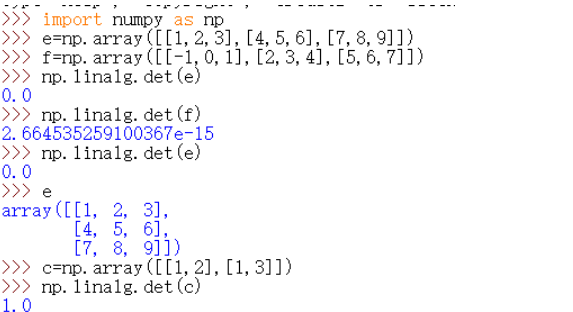在对e方阵求行列式时本机出现一定问题，不知为何显示的结果为0，而方阵f则没有问题

Python求逆矩阵/伴随矩阵
设A是数域上的一个n阶方阵，若在相同数域上存在另一个n阶矩阵B，使得： AB=BA=E。 则我们称B是A的逆矩阵，而A则被称为可逆矩阵。当矩阵A的行列式|A|不等于0时才存在可逆矩阵。
而伴随矩阵的定义为：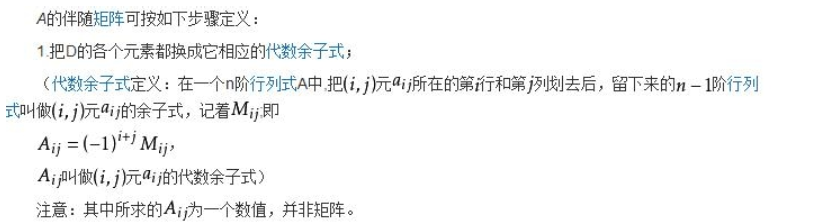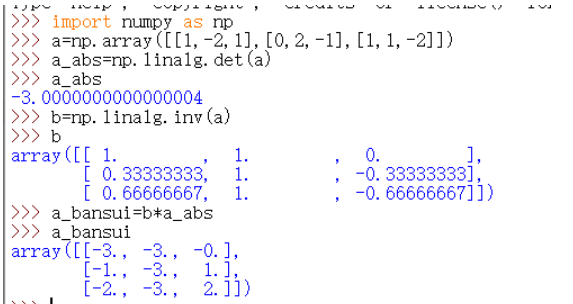Python解多元一次方程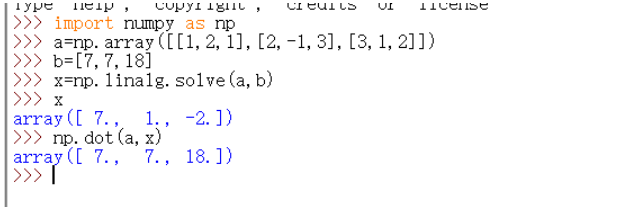使用Python求解多元一次方程的原理也是将多元方程组代入矩阵后求解，主要运用的方法还是换元

## 微分、梯度的含义

微分
由函数B=f(A)，得到A、B两个数集，在A中当dx靠近自己时，函数在dx处的极限叫作函数在dx处的微分，微分的中心思想是无穷分割。通常把自变量x的增量 Δx称为自变量的微分，记作dx，即dx = Δx。于是函数y = f(x)的微分又可记作dy = f’(x)dx。函数因变量的微分与自变量的微分之商等于该函数的导数。因此，导数也叫做微商。
几何意义：当Δx很小时，切线纵坐标的增量。

梯度
梯度的本意是一个向量（矢量），表示某一函数在该点处的方向导数沿着该方向取得最大值，即函数在该点处沿着该方向（此梯度的方向）变化最快，变化率最大（为该梯度的模）。

梯度下降法

梯度下降法求解回归方程的python代码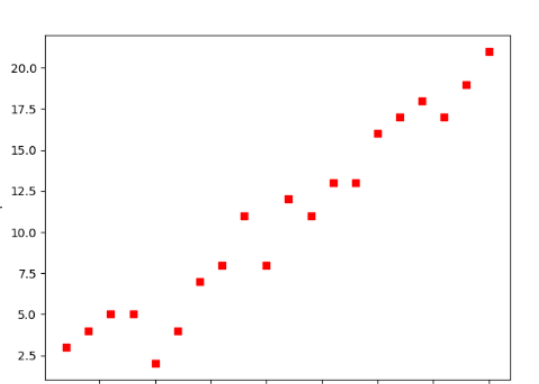为上图的点用梯度下降法求解拟合直线，具体代码如下

from numpy import *

# 数据集大小 即20个数据点
m = 20
# x的坐标以及对应的矩阵
X0 = ones((m, 1))  # 生成一个m行1列的向量，也就是x0，全是1
X1 = arange(1, m+1).reshape(m, 1)  # 生成一个m行1列的向量，也就是x1，从1到m
X = hstack((X0, X1))  # 按照列堆叠形成数组，其实就是样本数据
# 对应的y坐标
Y = array([
3, 4, 5, 5, 2, 4, 7, 8, 11, 8, 12,
11, 13, 13, 16, 17, 18, 17, 19, 21
]).reshape(m, 1)
# 学习率
alpha = 0.01

# 定义代价函数
def cost_function(theta, X, Y):
diff = dot(X, theta) - Y  # dot() 数组需要像矩阵那样相乘，就需要用到dot()
return (1/(2*m)) * dot(diff.transpose(), diff)

# 定义代价函数对应的梯度函数
diff = dot(X, theta) - Y
return (1/m) * dot(X.transpose(), diff)

# 梯度下降迭代
theta = array([1, 1]).reshape(2, 1)
theta = theta - alpha * gradient
return theta

print('optimal:', optimal)
print('cost function:', cost_function(optimal, X, Y))

# 根据数据画出对应的图像
def plot(X, Y, theta):
import matplotlib.pyplot as plt
ax = plt.subplot(111)  # 这是我改的
ax.scatter(X, Y, s=30, c="red", marker="s")
plt.xlabel("X")
plt.ylabel("Y")
x = arange(0, 21, 0.2)  # x的范围
y = theta + theta*x
ax.plot(x, y)
plt.show()

plot(X1, Y, optimal)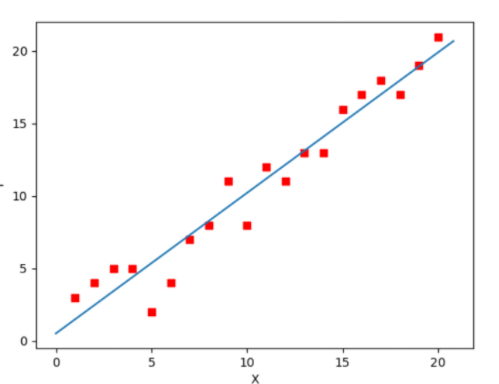拟合结果如上

展开全文人工智能
• ## python矩阵运算

万次阅读 2016-07-31 16:59:41
科学计算：Python VS. MATLAB(3)----线性代数基础  按：在介绍工具之前先对理论基础进行必要的回顾是很必要的。没有理论的基础，讲再多的应用都是空中楼阁。本文主要设涉及线性代数和矩阵论的基本内容。先回顾这...python
• python的numpy库提供矩阵运算的功能，因此我们在需要矩阵运算的时候，需要导入numpy的包。 1.numpy的导入和使用 from numpy import *;#导入numpy的库函数 import numpy as np; #这个方式使用numpy的函数时，需要以...
• python矩阵基本运算一、python矩阵操作二、python矩阵乘法三、python矩阵转置四、python求方阵的迹五、python方阵的行列式计算方法六、python求逆矩阵/伴随矩阵七、python解多元一次方程 一、python矩阵操作 1、引入...
• python矩阵运算第一次看见Python的运行感觉就让我想起了matlab，于是就上网嗖嗖他在矩阵方面的运算如何，如果不想安装Matlab那么大的软件，而你又只是想计算些矩阵，python绝对够用！尤其在Linux下太方便了Python...python矩阵计算
•numpy python
• 使用python和R进行科学计算，最为人所诟病的是它们的运算速度。然而，通过关联Intel MKL线性代数库，你可以使python和R达到和matlab类似的运算速度，并且自动使用闲置CPU核心进行并行。python r语言
• Python使用NumPy包完成了对N-维数组的快速便捷操作。使用这个包，需要导入numpy。SciPy包以NumPy包为基础，大大的扩展了numpy的能力。为了使用的方便，scipy包在最外层名字空间中包括了所有的numpy内容，因此只要...
• 1 python矩阵运算所需模块 import numpy as np import matplotlib.pyplot as plt import scipy.linalg as lg #scipy矩阵运算模块 2 定义矩阵和进行相关的矩阵运算 print(lg.inv(a)) #求取矩阵的逆矩阵 print(lg.det...python
• ## Python矩阵基本运算【numpy】

万次阅读 多人点赞 2021-03-31 16:37:34
python矩阵操作2. python矩阵乘法3. python矩阵转置4. python求方阵的迹5. python方阵的行列式计算方法6. python求逆矩阵/伴随矩阵7. python解多元一次方程 一、实验说明 实验环境 Anaconda + python3.6 + jupyter...python numpy
• ## python矩阵的基本运算

万次阅读 多人点赞 2021-04-05 15:43:19
文章目录一、python矩阵操作二、python矩阵乘法三、python矩阵转置四、python求方阵的迹五、python方阵的行列式计算方法六、...通常情况下，使用二维数组代替矩阵来进行矩阵运算，可见矩阵和数组基本上都可以 加减法.
• 机器学习数学基础之 Python 矩阵运算（人工智能与机器学习）一、Python 矩阵基本运算1. Python 矩阵操作2. Python 矩阵乘法3. 矩阵的转置4. Python 求方阵的迹5. Python 方阵的行列式计算方法6. Python 求逆矩阵/...
• 当两个较大的 (e.g., 10000*10000 维）ndarray 做运算(加法，or 乘法）时，很容易出现这样的结果. 解决办法： 大多数情况下，这种大矩阵都是稀疏的。尽可能地利用稀疏计算的方式，例如稀疏矩阵，或者只计算非 0 ...
• python进行矩阵运算的方法： 1、矩阵相乘 >>>a1=mat([1,2]); >>>a2=mat([,]); >>>a3=a1*a2 #1*2的矩阵乘以2*1的矩阵，得到1*1的矩阵 >>> a3 matrix([]) 2、矩阵对应元素相乘 >>>a1=mat([1,1]); >>>a2=mat......